﻿ 再平衡：去杠杆与稳增长——基于存量－流量一致模型的分析
 财经研究2018, Vol. 44Issue (10): 4-230

#### 文章信息财经研究2018年44卷第10期

Chen Dafei, Shao Yu, Yang Xiaohai.

Rebalance: deleveraging and stabilizing economic growth — based on an analysis of the stock-flow consistent model

Journal of Finance and Economics, 2018, 44(10): 4-23.

### 文章历史《财经研究》
2018第44卷第10期

1. 东方证券，上海 200010;
2. 清华大学 国家金融研究院，北京 100083

Rebalance: Deleveraging and Stabilizing Economic Growth — Based on an Analysis of the Stock-Flow Consistent Model
Chen Dafei1, Shao Yu1, Yang Xiaohai2
1. Orient Securities，Shanghai 200010，China;
2. National Institute of Financial Research，Tsinghua University，Beijing 100083，China
Summary: Dealing with financial risks has become a focus of China’s economic and financial policies. High leverage and structural imbalance of leverage are the main sources of risks in contemporary China, and the latter one is the core issue. According to the data from BIS, up to June 2017, China’s macro leverage (total debt/GDP) was 255.9%, which was above the emerging market average 190%, and the U.S. 249.5%. In terms of the structure, China’s non-financial sector exhibits a leverage ratio of 163.4%, which is ranked first among all major economies. This figure is well above the euro zone, Japan and the U.S. at 103.4%, 102.1% and 73.3% respectively. China’s ratio is also higher than that of emerging market economies such as India. The leverage ratios of China’s household sector and the government sector are 46.8% and 45.7% respectively. Although these figures are relatively low, the residential sector’s leverage is escalating rapidly. Therefore, macro-leverage and structural imbalance are serious challenges. It not only increases financial risks, but also drags down the real/tangible economy. Thus, reducing debt leverage while stabilizing economic growth at the same time is a focus of economic and financial policies.　　The innovation of this paper is embodied in methodology. In reality, the economy is often in " suboptimal equilibrium” rather than in " optimal equilibrium”, so correcting a " distortion” does not necessarily bring about efficiency. As a result, the local equilibrium analysis cannot fully depict the macro-economic operation. Due to assumptions such as profit maximization etc., mainstream methodology represented by the DSGE model is controversial after the 2008 financial crisis. Just like Koo (2003) pointed out, " debt minimization” is the objective of the economic agents in the " Yin” stage of the economic cycle.　　This study constructs a five-sector stock-flow consistent model to analyze heterogeneous effects on deleveraging and stabilizing the economic growth of various monetary policies, fiscal policies and enterprise micro-level measures (or portfolios of the measures). The SFC model integrates the transaction behavior of macroeconomic departments into a unified dynamic framework and considers the consistency of stock and flow, which is helpful to overcome the deficiencies of the local equilibrium analysis. Moreover, no optimization condition is required for the SFC model.　　Simulation results show that the contractionary monetary policy will increase the leverage of governments and enterprises, decrease household leverage, and exert a mild negative effect on macro-economy; the increasing growth rate of government expenditure and the decreasing personal income tax rate will both have a win-win effect on deleveraging and stabilizing economic growth, but with a more effective deleveraging result for former measure. The latter one can balance deleverage and economic growth in a more efficient way. Equity financing has an apparent effect on enterprise deleveraging with a mild negative effect on economic growth; transitioning from the investment-driven mode to the consumption-driven mode of economic development will in favor of deleveraging for the government and enterprise sectors while balancing economic growth depends on different portfolios of policies. For enterprise micro-level measures, decreasing the dividend payout ratio for example, will have different effects on deleveraging for different macro-sectors.　　Deleveraging is a comprehensive task. The leverage does not vanish out of thin air, so it needs to be analyzed both from the macroscopic and structural perspectives. The influence of the policies on the numerator and the denominator of the same equation needs to be considered at the same time. Taking monetary policies as an example, the tightening of monetary policies, on the one hand, will reduce debt growth, that is, to prevent the augmentation of leverage. Nevertheless, it will also affect the economic growth rate. Therefore, the combined influence does not necessarily reduce the leverage. The final effect depends on the relative changes in the numerator and the denominator. As the case for China, which has high debt stocks and leverage, tighter monetary policies would aggravate the interest burden on highly leveraged sectors and increase the probability of some companies conducting speculating and Ponzi financing.
Key words: deleveraging    stabilizing economic growth    stock-flow consistent model

Fisher（1933）在探讨经济周期问题时认为，繁荣与萧条周期性更替的主要扰动因素是过度负债和通缩，它不仅增加了金融风险，也拖累了实体经济。但他同时也强调，债务−通缩循环是可以通过再通胀和一些稳定政策来预防的。2017年中央经济工作会议强调，未来几年“防范重大风险”是“三大攻坚战”之一，而杠杆问题是连接实体部门与金融部门的“枢纽”。

Minsky（1992）认为，宏观经济的运行可以用一系列相互联系的资产负债表与现金流量表来描述。该方法的一个主要代表是基于资产负债表（Balance Sheet-Stock TableBSST）和现金流量表（Transaction-Flow TableTFT）约束的存量−流量一致（Stock-Flow ConsistentSFC）模型（Godley，1996；Godley和Lavoie，2007）。主流方法将宏观模型建立在理性行为人最优化选择的基础上，将货币看作一层“面纱”，将商业银行仅看作借贷的媒介，或将金融体系的运作排除在模型之外。而SFC方法从“环流”的视角看经济运行，将货币看作交易媒介与记账手段，将商业银行看作信贷创造的主体，区分了不同的金融资产，采用部门分析法将金融体系与实体经济纳入统一的框架下研究，而且没有理性人和信息约束。SFC模型在国际上已被广泛用于分析金融危机、财政和货币政策、流动性偏好、产能利用率和影子银行等问题，而在中国，这方面的研究相对欠缺。本文旨在构建一个基准模型，分析各种政策的去杠杆（或移杠杆）和稳增长效应。

（一）资产负债−存量表

 家庭 企业 商业银行 政府 中央银行 总计 1. 物质资本 +K +K 2. 存货 +IN +IN 3. 基础货币 +MBh +MBb −MB 0 4. 存款 +Dh −D 0 5. 贷款 −Lh −Lf +L 0 6. 国库券 +Bh +Bb −B +Bcb 0 7. 长期国债 +PBLBLh −PBLBL 0 8. 股票 +PEEh −PEE 0 9. 净资产 −Vh −Vf 0 −Vg 0 −(K+IN) 10. 总计 0 0 0 0 0 0

（二）交易−流量表

 家庭 企业 商业银行 政府 中央银行 总计 资本账户 经常账户 资本账户 经常账户 资本账户 经常账户 1. 消费 −C +C 0 2. 投资 +（I+ΔIN） −（I+ΔIN） 0 3. 政府支出 +G −G 0 4. 税收 −T +T 0 5. 工资 +WB −WB 0 6. 库存融资成本 ${ - {r_{L - 1}}\Delta I{N_{ - 1}}}$ ${ + {r_{L - 1}}\Delta I{N_{ - 1}}}$ 0 7. 存款利息 ${ + {r_{D - 1}}{D_{ - 1}}}$ ${ - {r_{D - 1}}{D_{ - 1}}}$ 0 8. 贷款利息 ${ - {r_{L - 1}}{L_{h - 1}}}$ ${ - {r_{L - 1}}({L_{f - 1}} - I{N_{ - 1}})}$ ${ + {r_{L - 1}}{L_{ - 1}}}$ 0 9. 国库券利息 ${ + {r_{B - 1}}{B_{h - 1}}}$ ${ + {r_{B - 1}}{B_{b - 1}}}$ ${ - {r_{B - 1}}{B_{ - 1}}}$ ${ + {r_{B - 1}}{B_{cb - 1}}}$ 0 10. 长期国债利息 ${ + {r_{BL - 1}}B{L_{ - 1}}}$ ${ - {r_{BL - 1}}B{L_{ - 1}}}$ 0 11. 企业利润 +FDf −Ff +REf 0 12. 银行利润 +Fb −Fb +Fcb −Fcb 0 13. Δ 基础货币 −ΔMBh −ΔMBb +ΔMBcb 0 14. Δ 存款 −ΔDh +ΔD 0 15. Δ 贷款 +ΔLh +ΔLf −ΔL 0 16. Δ 国库券 −ΔBh −ΔBb +ΔB −ΔBcb 0 17. Δ 长期国债 −ΔBLhPBL +ΔBLPBL 0 18. Δ 股票 −ΔEhPE +ΔEhPE 0 19. 总计 0 0 0 0 0 0 0 0 0

（三）SFC模型构建

SFC模型中的方程主要分为约束方程和行为方程两类。下文将分部门阐述方程的构建及其含义。除了利率变量外，小写字母表示真实值，大写字母表示名义值，上标e表示期望值，上标T表示目标值，下标-1表示前期值。

1. 企业

（1）生产与投资

 1. ${y = {s^e} + \left( {i{n^e} - i{n_{ - 1}}} \right)}$ 10. ${\pi = \displaystyle\frac{{P - {P_{ - 1}}}}{{{P_{ - 1}}}}}$ 2. ${{s^e} = \beta s + \left( {1 - \beta } \right){s_{ - 1}}\left( {1 + g{r_{pr}}} \right)}$ 11. ${i = \left( {g{r_k} + {\delta _k}} \right) {k_{ - 1}}}$ 3. ${i{n^e} = i{n_{ - 1}} + \gamma \left( {i{n^T} - i{n_{ - 1}}} \right)}$ 12. ${s = c + i + g}$ 4. ${i{n^T} = {\sigma ^T} {s^e}}$ 13. ${S = s P}$ 5. ${in = i{n_{ - 1}} + \left( {y - s} \right)}$ 14. ${IN = in \times UC}$ 6. ${k = {k_{ - 1}} \left( {1 + g{r_k}} \right)}$ 15. ${I = i P}$ 7. ${g{r_k} = {\gamma _o} + {\gamma _u} u - {\gamma _r} r{r_L}}$ 16. ${K = k P}$ 8. ${u = \displaystyle\frac{y}{{{k_{ - 1}}}}}$ 17. ${Y = s P + \Delta in \times UC}$ 9. ${r{r_L} = \displaystyle\frac{{\left( {1 + {r_L}} \right)}}{{\left( {1 + \pi } \right)}} - 1}$ 18. ${UC = \displaystyle\frac{{WB}}{y}}$

（2）工资与就业

 19. ${{w^T} = {\Omega _0} + {\Omega _1} pr + {\Omega _2} \left[ {ER + {z_1}\left( {1 - ER} \right) + {z_2} Top - {z_3} Bot} \right]}$ 　 ${{z_1} = 1\;\;iff\;\;1 - Bot \leqslant ER \leqslant 1 + Top}$ 　 ${{z_2} = 1\;\;iff\;\;ER > 1 + Top}$ 　 ${{z_3} = 1\;\;iff\;\;ER < 1 - Bot}$ 20. ${ER = \displaystyle\frac{{{N_{ - 1}}}}{{{N_{fe - 1}}}}}$ 21. ${pr = p{r_{ - 1}} \left( {1 + g{r_{pr}}} \right)}$ 22. ${W = {W_{ - 1}} + {\Omega _3} \left( {{\omega ^T} {P_{ - 1}} - {W_{ - 1}}} \right)}$ 23. ${WB = N \times W}$ 24. ${N = {N_{ - 1}} + {\Omega _4} \left( {{N^T} - {N_{ - 1}}} \right)}$ 25. ${{N^T} = \displaystyle\frac{y}{{pr}}}$

（3）定价

 26. ${p = \left( {1 + \varphi } \right) NHUC}$ 27. ${NHUC = \left( {1 - {\sigma ^N}} \right) NUC + {\sigma ^N} \left( {1 + {r_L}} \right) NU{C_{ - 1}}}$ 28. ${NUC = \displaystyle\frac{W}{{pr}}}$

（4）利润及分配

 29. ${{F_f} = S + \Delta IN - WB - {r_{L - 1}} {L_{fd - 1}}}$ 34. ${{r_{DY}} = \displaystyle\frac{{F{D_f}}}{{{E_{s - 1}} {P_{E - 1}}}}}$ 30. ${{L_{fd}} = {L_{fd - 1}} + I + \Delta IN - R{E_f} - \Delta {E_s} {P_E}}$ 35. ${BU{R_f} = \displaystyle\frac{{{r_{L - 1}} {L_{fd - 1}}}}{{{F_f}}}}$ 31. ${F{D_f} = {\varphi _D} {F_{f - 1}}}$ 36. ${Lev\_{y_f} = \displaystyle\frac{{{L_{fd}}}}{Y}}$ 32. ${R{E_f} = {F_f} - F{D_f}}$ 37. ${Lev\_{e_f} = \displaystyle\frac{{{L_{fd}}}}{{{E_s} {P_E}}}}$ 33. ${{E_s} = {E_{s - 1}} + \left( {1 - \varphi _{RE}^T} \right) \displaystyle\frac{{{I_{ - 1}}}}{{{P_E}}}}$

2. 居民

（1）收入与消费

 38. ${YP = WB + F{D_f} + {F_b} + {r_{D - 1}} {D_{hd - 1}} + {r_{B - 1}} {B_{hd - 1}} + {r_{BL - 1}} B{L_{d - 1}}}$ 39. ${Y{D_r} = YP - T - {r_{L - 1}} {L_{hd - 1}}}$ 47. ${nl = \displaystyle\frac{{NL}}{P}}$ 40. ${T = \theta \times YP}$ 48. ${NL = \left( {{\varepsilon _1} - {\varepsilon _2} r{r_L}} \right) YP - REP}$ 41. ${Y{D_{HS}} = Y{D_r} + CG}$ 49. ${yd_r^e = {\varepsilon _3} y{d_r} + \left( {1 - {\varepsilon _3}} \right) y{d_{r - 1}} \left( {1 + g{r_{pr}}} \right)}$ 42. ${CG = \Delta {P_E} {E_{hd - 1}} + \Delta {P_{BL}} B{L_{d - 1}}}$ 50. ${y{d_r} = \displaystyle\frac{{Y{D_r}}}{P} - \displaystyle\frac{{\pi {V_{ - 1}}}}{P}}$ 43. ${V = {V_{ - 1}} + Y{D_{HS}} - C}$ 51. ${REP = {\varepsilon _4} {L_{hd - 1}}}$ 44. ${v = \displaystyle\frac{V}{P}}$ 52. ${{L_{hd}} = {L_{hd - 1}} + NL}$ 45. ${C = c \times P}$ 53. ${BU{R_h} = \displaystyle\frac{{REP + {r_{L - 1}} {L_{hd - 1}}}}{{YP}}}$ 46. ${c = {\alpha _1} \left( {yd_r^e + nl} \right) + {\alpha _2} {v_{ - 1}}}$ 54. ${Lev\_{v_h} = \displaystyle\frac{{{L_{hd}}}}{V}}$

（2）资产组合选择

 55. ${\displaystyle\frac{{{B_{hd}}}}{{{V_{FA - 1}}}} = {\lambda _{20}} - {\lambda _{21}} {r_D} + {\lambda _{22}} {r_B} - {\lambda _{23}} {r_{DY}} - {\lambda _{24}} {r_{BL}} + {\lambda _{25}} \left( {\displaystyle\frac{{Y{D_{r - 1}}}}{{{V_{FA - 1}}}}} \right)}$ 56. ${\displaystyle\frac{{{P_{BL}} B{L_d}}}{{{V_{FA - 1}}}} = {\lambda _{30}} - {\lambda _{31}} {r_D} - {\lambda _{32}} {r_B} + {\lambda _{33}} {r_{DY}} - {\lambda _{34}} {r_{BL}} + {\lambda _{35}} \left( {\displaystyle\frac{{Y{D_{r - 1}}}}{{{V_{FA - 1}}}}} \right)}$ 57. ${\displaystyle\frac{{{P_E} {E_{hd}}}}{{{V_{FA - 1}}}} = {\lambda _{40}} - {\lambda _{41}} {r_D} - {\lambda _{42}} {r_B} - {\lambda _{43}} {r_{DY}} + {\lambda _{44}} {r_{BL}} + {\lambda _{45}} \left( {\displaystyle\frac{{Y{D_{r - 1}}}}{{{V_{FA - 1}}}}} \right)}$ 58A. ${\displaystyle\frac{{{D_{hd}}}}{{{V_{FA - 1}}}} = {\lambda _{10}} + {\lambda _{11}} {r_D} - {\lambda _{12}} {r_B} - {\lambda _{13}} {r_{DY}} - {\lambda _{14}} {r_{BL}} + {\lambda _{15}} \left( {\displaystyle\frac{{Y{D_{r - 1}}}}{{{V_{FA - 1}}}}} \right)}$ 58. ${{D_{hd}} = {V_{FA}} - {B_{hd}} - {P_{BL}} B{L_d} - {P_E} {E_{hd}}}$ 59. ${{V_{FA}} = V + {L_{hd}} - M{B_{hd}}}$ 60. ${M{B_{hd}} = {\lambda _c} C}$

3. 政府：支出、赤字与债务

 61. G=g×P 65. BLs=BLhd 62. g=g-1（1+grg） 66. GD=Bs+BLsPBL 63. GFD=G+rB-1Bs-1+rBL-1BLs-1−T−Fcb 67. ${{P_{BL}} = \displaystyle\frac{1}{{{r_{BL}}}}}$ 64. Bs=Bs-1+GFD−ΔBLs-1PBL 68. ${BU{R_g} = \displaystyle\frac{{{r_{B - 1}} {B_{s - 1}} + {r_{BL - 1}} B{L_{s - 1}}}}{{T + {F_{cb}}}}}$

4. 中央银行：基准利率与货币发行

 69. Fcb=rB-1Bcbd-1 72. Bcbd=MBs 70. Bcbd=Bs−Bhd−Bbd 73. ${r_B} = \overline {{r_B}}$ 71. MBs=MBhd+MBbd 74. rBL=rB+addBL

5. 商业银行：存贷款利率及利润

 75. Fb=rL-1（Lfd-1+Lhd-1）+rB-1Bbd-1−rD-1Ds-1 78. Ds=Dhd 76. Bbd=Ds−Lfd−Lhd−MBbd 79. ${r_D} = \overline {{r_D}}$ 77. MBbd=ρDs 80. rL=rD+addL

（四）参数设定与稳态解

SFC模型中，参数设定一般有估计和校准两种方法。估计法基于时间序列数据对参数进行估计。以利维（Levy）研究所为代表的模型被称为完全实证模型（Fully empirical models），Godley和Zezza（1986）最早采用该方法，构建了一个简化的SFC模型，以丹麦为例进行了模拟。该方法的问题在于，预测的基本前提是参数保持不变，而这正是卢卡斯批判的主要内容。与之不同，利默里克（Limerick）模型（Kinsella和Tiou-Tagba Aliti，2012）仅基于已有的存量数据对关键方程的参数进行估计，其他参数则基于校准得到。该模型的主要用途并非预测，而是对历史进行模拟，如模拟某项政策的实施效果等。

SFC模型的求解有两种途径。对于相对简单的模型，可以求得解析解，并通过比较静态分析来研究变量的动态特征和相互关系，或者通过代入参数与变量初始值，获得稳态解。为了获得解析解，要求构建简化的模型，但这样做必须省略某些现实特征，有损模型的完整性。对于相对复杂的模型，一般的做法是利用计算机模拟求得稳态解。本文的模型比较复杂，因而直接利用计算机模拟求得稳态解，并以此为参照系，通过重新设定单个或多个参数来考察模型的脉冲响应。

（一）货币政策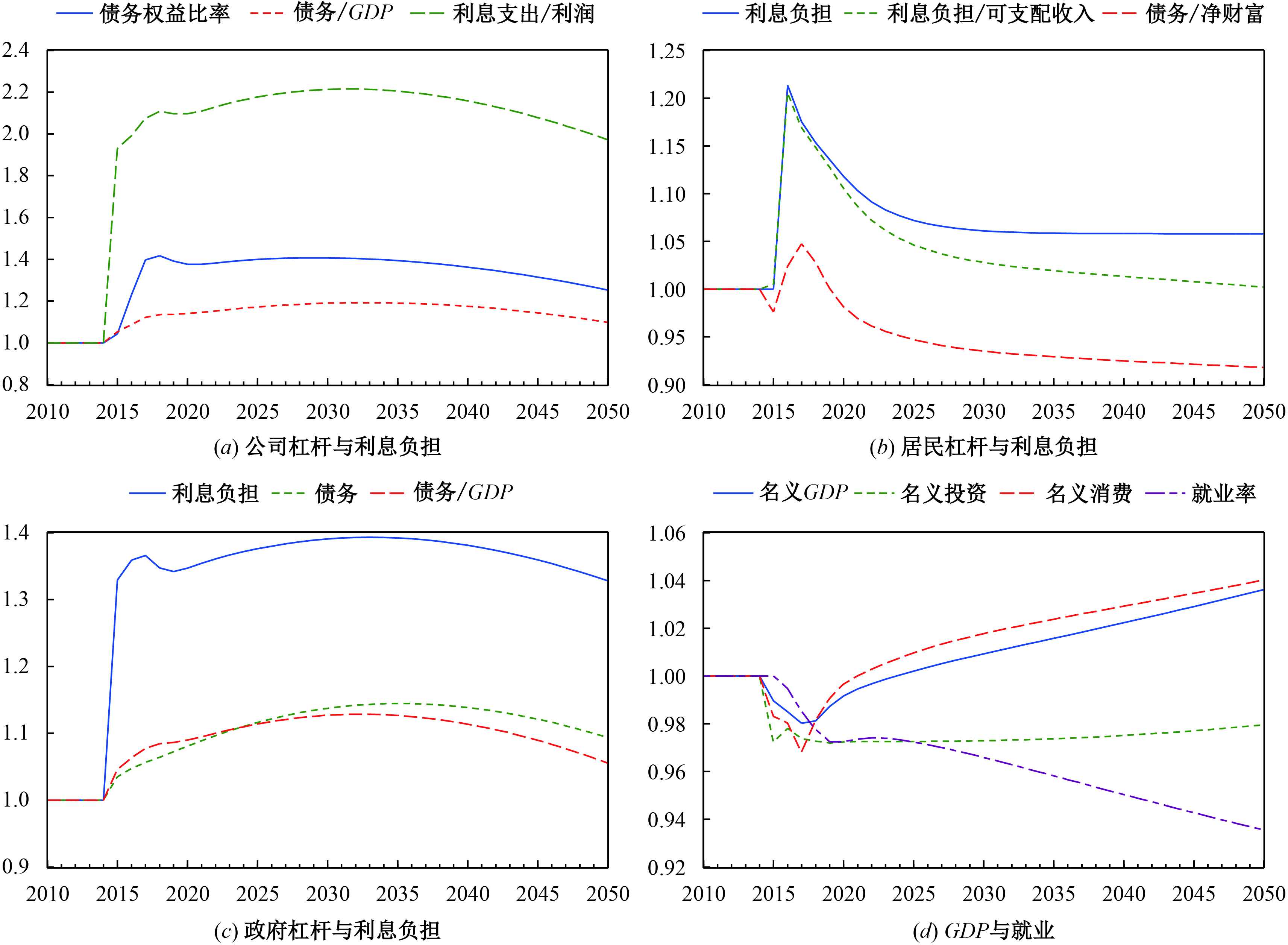图 1 紧缩性货币政策对各部门杠杆与宏观经济的影响

（二）财政政策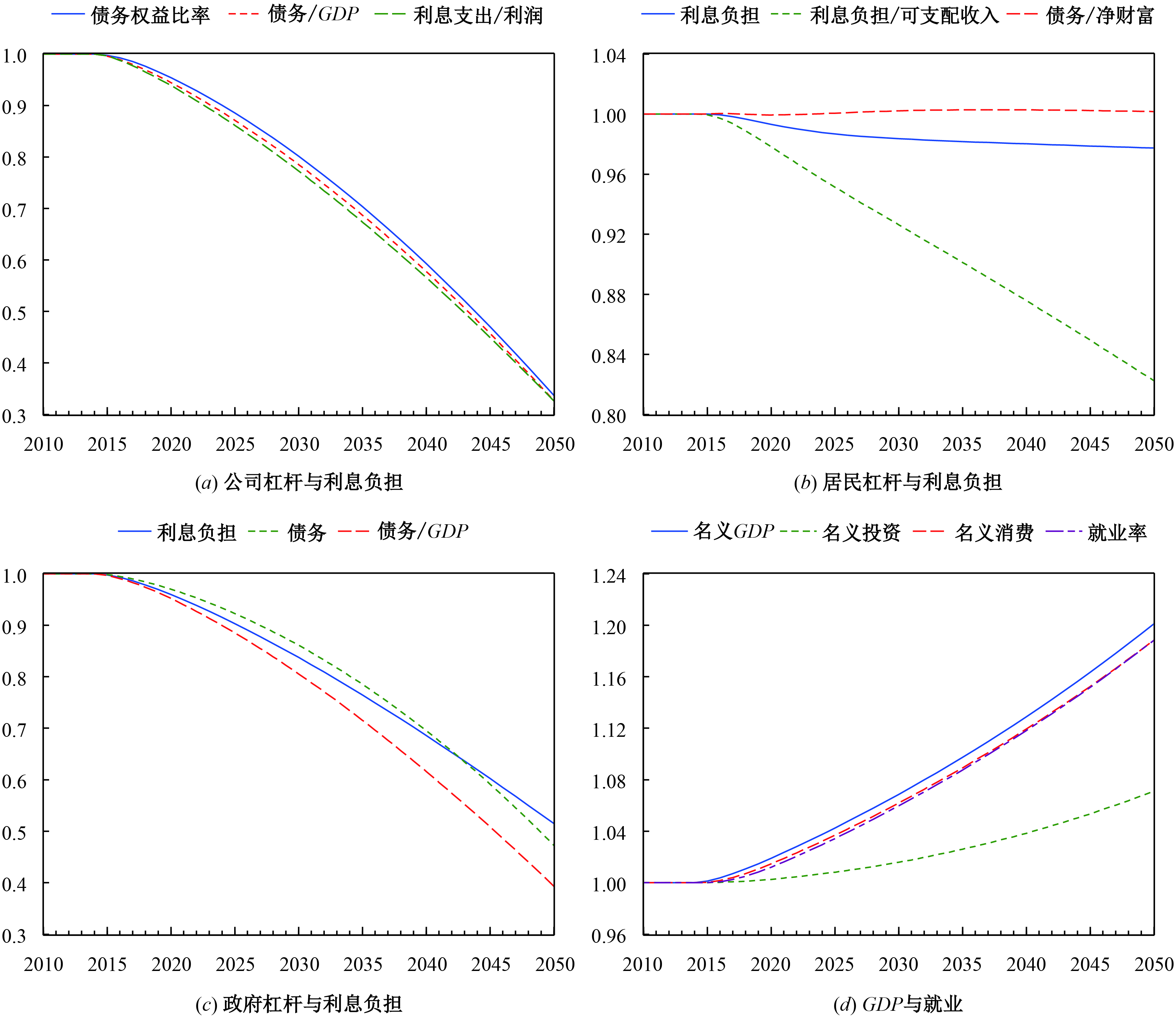图 2 提高政府支出增速对各部门杠杆与宏观经济的影响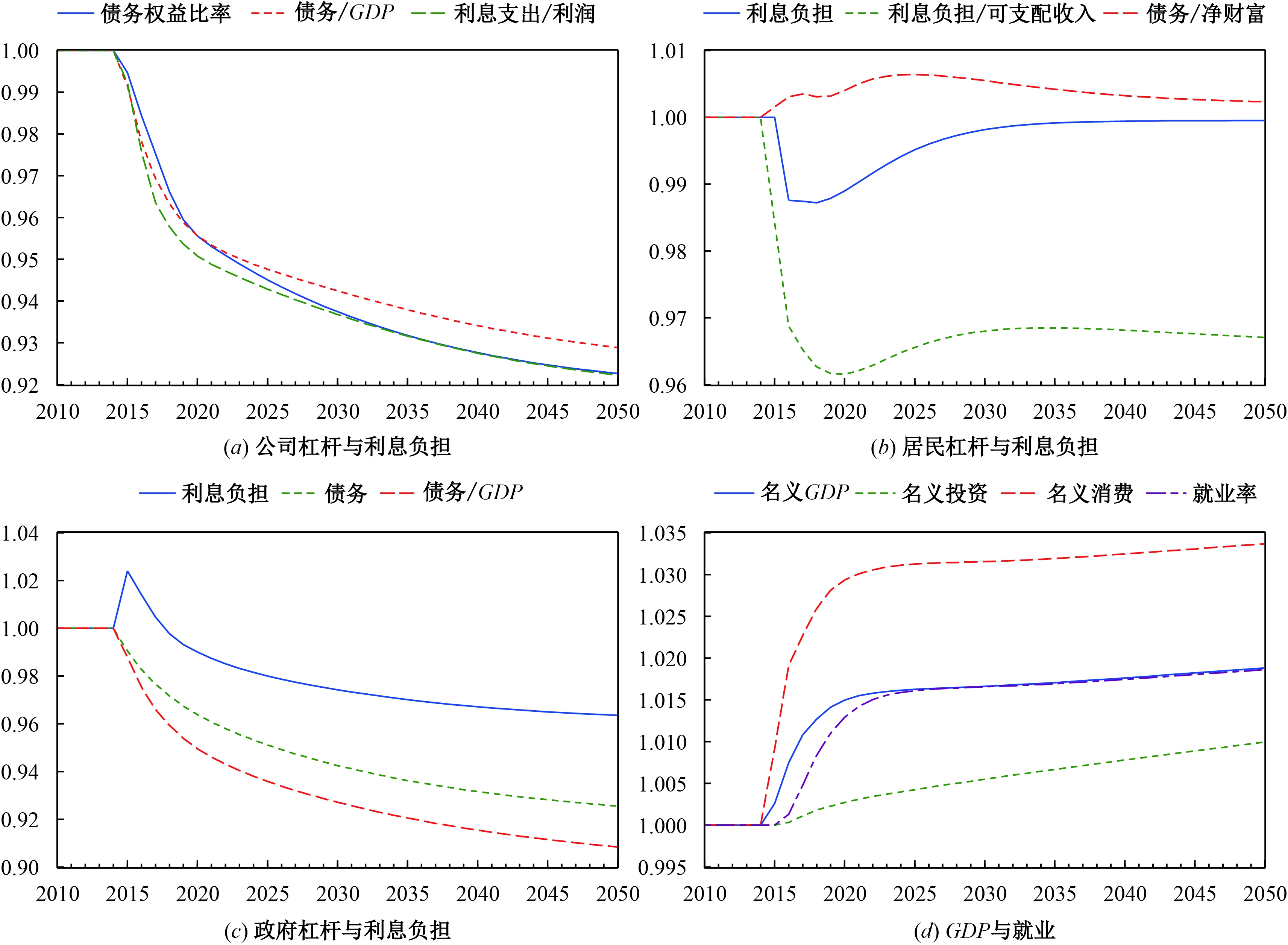图 3 减税对各部门杠杆与宏观经济的影响

（三）股权融资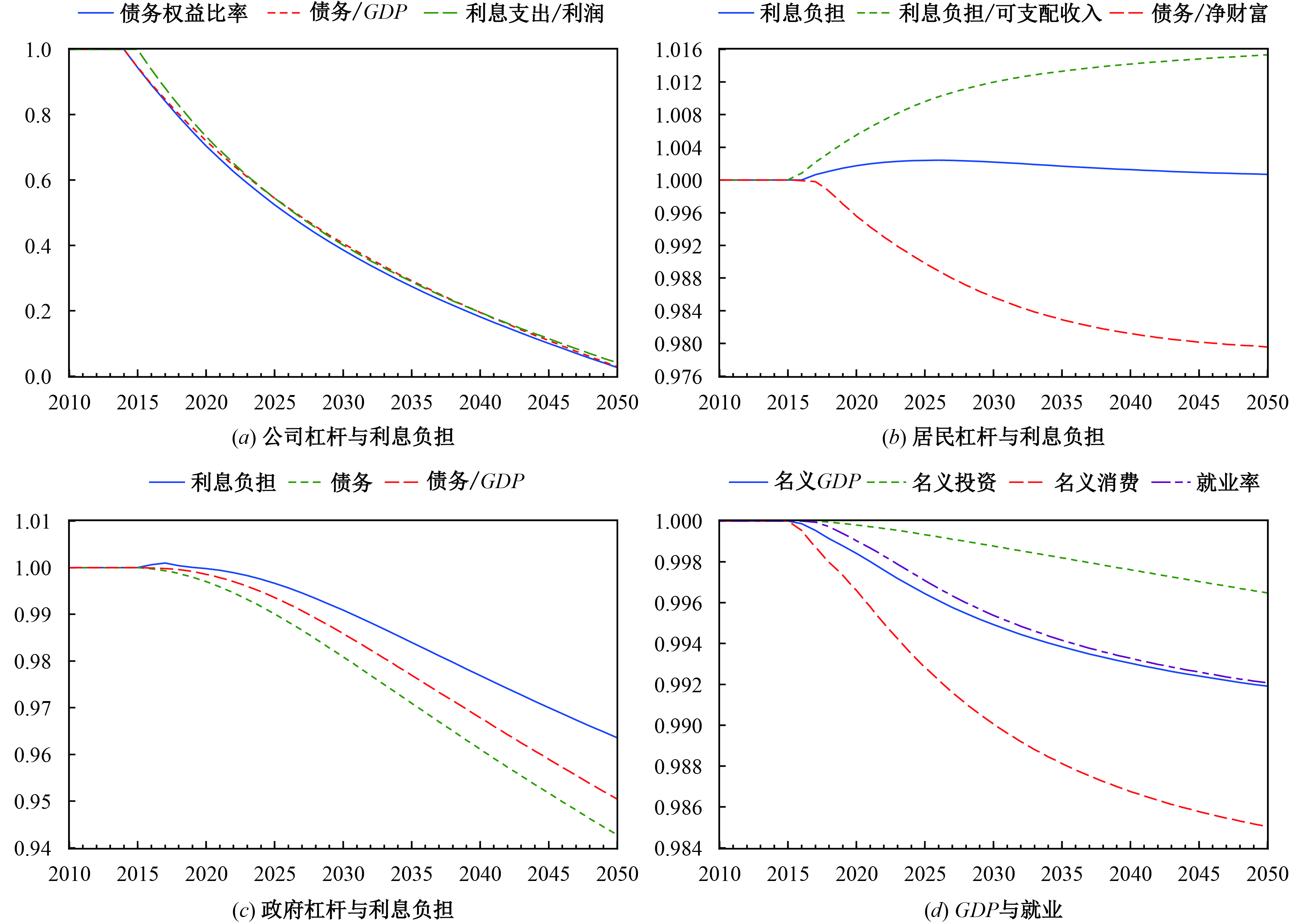图 4 股权融资对各部门杠杆与宏观经济的影响

（四）经济增长模式转变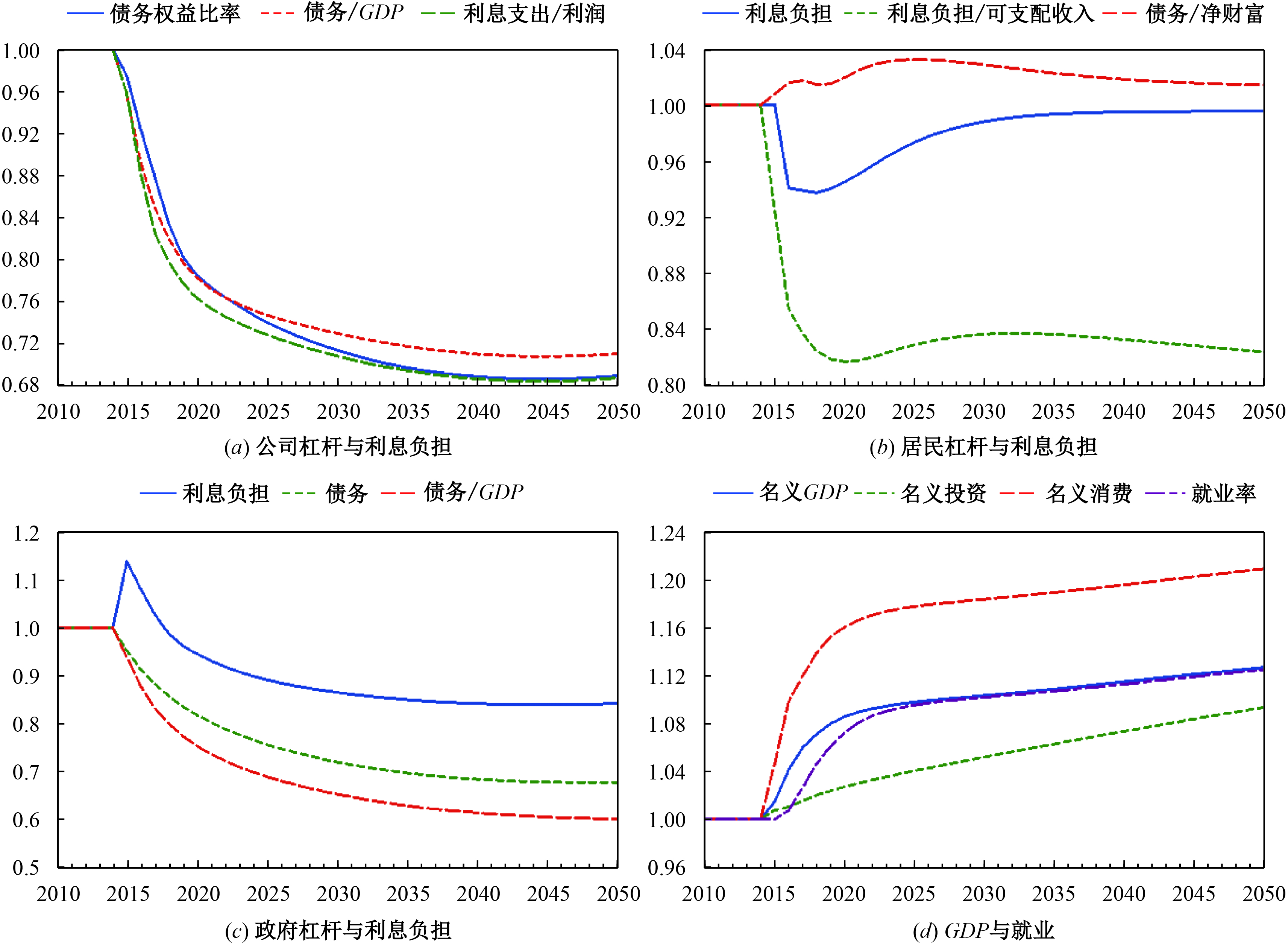图 5 投资驱动向消费驱动转变（减税）对各部门杠杆与宏观经济的影响

（五）去杠杆的微观视角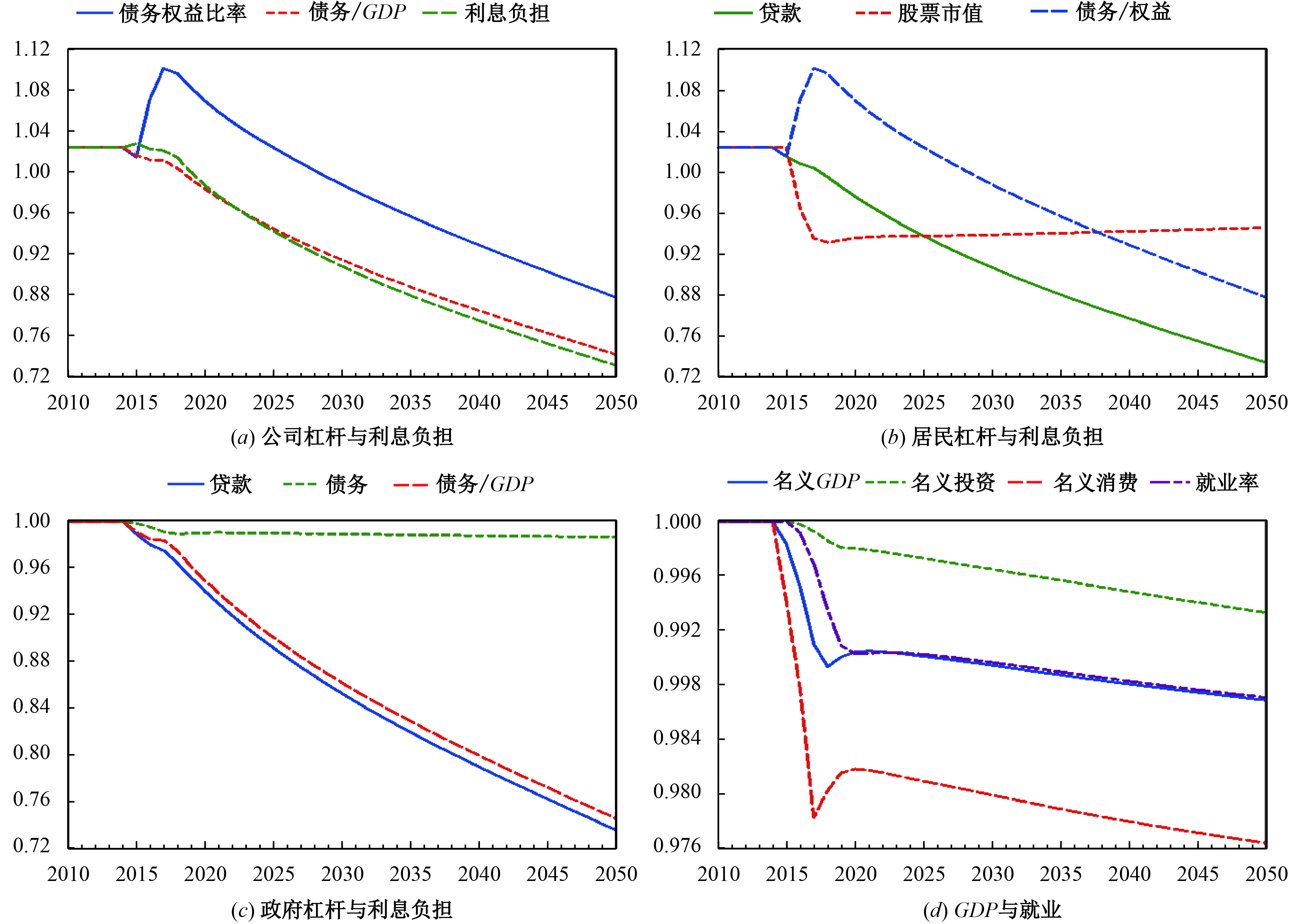图 6 降低派息率对各部门杠杆与宏观经济的影响

 参数 含义 数值 校准依据 β 预期销售额方程中当期真实销售额的权重系数 0.5 Godley和Lavoie(2007) grpr 劳动生产率增长率 0.093 2008—2016年均值（世界劳工组织） γ 预期库存调整系数 0.15 Godley和Lavoie(2007) σT 目标库存占预期销售的比例 0.09 工业企业库存率历史均值 γo 自发固定资本投资增速 0.025 Lavoie和Zhao（2010） γu 固定资本投资增速对产能利用率的敏感系数 0.05 Godley和Lavoie(2007) γr 固定资本投资增速对真实利率的敏感系数 1 Lavoie和Zhao（2010） δk 固定资本折旧率 0.125 文献中资本年折旧率一般在0.1和0.15之间，本文取均值 Ω0 目标工资率函数中的常数项 −0.21 Godley和Lavoie(2007) Ω1 目标工资率对劳动生产率的敏感系数 1 Godley和Lavoie(2007) Ω2 目标工资率对就业率的敏感系数 2 Godley和Lavoie(2007) Ω3 名义工资率调整系数 0.46 Godley和Lavoie(2007) Ω4 就业人数调整系数 0.6 Godley和Lavoie(2007) Nfe-1 总劳动力人口 83.18 由就业人数与失业率序列推算得到 φ 价格加成比例 0.1 根据陈昆亭等（2004）以及黄志刚和陈晓杰（2010）校准 σN 历史的单位成本调整系数 0.17 Godley和Lavoie(2007) φD 派息率 0.35 根据上市公司年派息率均值校准 φRET 留存收益率 0.65 根据上市公司留存收益率均值校准 θ 个人收入所得税 0.25 个人收入所得税率中位数 ε1 居民净贷款对个人收入的敏感系数 0.074 AR（1）回归系数 ε2 居民净贷款对真实利率的敏感系数 0.4 AR（1）回归系数 ε3 预期真实可支配收入调整的权重系数 0.5 Godley和Lavoie(2007) ε4 贷款偿还额占贷款存量的比例 0.25 根据贷款存量及新增量数据测算 α1 预期真实可支配收入的边际消费倾向 0.8 Lavoie和Zhao（2010） α2 真实财富的边际消费倾向 0.05 Lavoie和Zhao（2010）附图 1 政府支出、央行利润与税收附图 2 居民可支配收入与资产组合管理

① 柳欣等（2012，2013）曾对SFC方法做过简要介绍，而建立五部门SFC模型并进行数值模拟的研究还是空白。

h表示居民，f表示非金融企业，b表示商业银行，g表示政府，cb表示中央银行；E表示企业发行的股票，BL表示政府长期债券。

③ 净资产项均为负值，因为可以将其理解为“所有者权益”，在会计报表中与负债记在同列。

PBL为长期国债价格，BL为国债存量。PE为股票市场价格，Eh为股票份额。

⑤ 对我国而言，国债的购买主体是券商资管、专户和私募等，由于本文并没有对大金融行业进行细分，这里将居民看作长期国债的最终拥有人。

⑥ 长期国债与股票账面价值的变动有两个原因：一是市场价格的变动，二是存量的变动。表2中的价值重估是指第二种原因所引起的账面价值变动。它与一个部门的收支行为是串联起来的，如果生产循环和金融循环表示某部门收大于支，则它将表现为该部门某项（或几项）金融资产数量的增加（假定其他不变）。价格变动所引起的变化将在后文中由资本利得方程表示。

⑦ 方程55−方程58A的参数设置需满足垂直约束与对称约束，具体可参见Godley和Lavoie（2007）第5章。

⑧ 下文的脉冲响应均以稳态为参照系。

⑨ 受篇幅限制，除了一些预期之外的结论，各种政策冲击的脉冲响应图都只有4幅，分别为企业、居民和政府的利息负担和债务杠杆及其对宏观经济的冲击。

⑩ 融资优序理论认为企业的融资偏好排序是内源融资（留存收益）、股权融资和债务融资。但肖泽忠和邹宏（2008）认为我国上市公司具有显著的股权融资偏好。

⑪ 在脉冲分析时需要更加关注短期效应，这是因为如“卢卡斯批判”所言，长期来看，经济结构和政策等都会变化，参数也会发生变化。因此，本文分析长期效应仅提供一个参照系。

⑫ 我们还考察了税率一次性降到25%时的脉冲响应，基本结论保持不变，但冲击效应变大。可见，减税的去杠杆效应随减税力度的增大而增强。

⑬ 读者无需在意2050年的债务杠杆几乎为0的情形，在内源融资与股权融资能够满足投资需求时，企业不存在债务融资需求，因而债务杠杆趋向于0，这与融资优序理论是一致的。

⑭ 在现有的模型中，考察企业的融资选择行为对杠杆的影响，还可以通过降低股息率φD来增加留存收益。基本结论与图4一致。

⑮ 本文还模拟了企业投资增速下降和居民部门消费倾向上升的情形，去杠杆的效果更加明显，但对经济增长有一定的负面影响。

⑯ 由于函数的非线性特征，削减工资的脉冲响应图呈现出非平滑的特征。由于数字变化非常小，基本可以忽略其影响。为节省篇幅，这里未展示。

⑰ 在20世纪70年代，诺贝尔奖得主Tobin在耶鲁大学的研究团队及Godley在剑桥大学的团队都是SFC模型的研究中心。只是新凯恩斯学派和理性预期学派兴起以后，这种方法逐渐被遗忘了。

  陈昆亭, 龚六堂, 邹恒甫. 什么造成了经济增长的波动, 供给还是需求: 中国经济的RBC分析[J]. 世界经济, 2004(4): 3–11.  胡志鹏. " 稳增长”与" 控杠杆”双重目标下的货币当局最优政策设定[J]. 经济研究, 2014(12): 60–71.  黄志刚, 陈晓杰. 人民币汇率波动弹性空间评估[J]. 经济研究, 2010(5): 41–54.  李杨, 张晓晶, 常欣, 等. 中国国家资产负债表2015——杠杆调整与风险管理[M]. 北京: 中国社会科学出版社, 2015.  柳欣, 赵雷, 吕元祥. 我国经济增长中的需求结构失衡探源——基于存量−流量均衡的分析视角[J]. 经济学动态, 2012(7): 57–63.  柳欣, 赵雷, 吕元祥. 宏观经济学的存量流量一致模型研究述评[J]. 经济学动态, 2013(12): 15–23.  吕炜, 高帅雄, 周潮. 投资建设性支出还是保障性支出——去杠杆背景下的财政政策实施研究[J]. 中国工业经济, 2016(8): 5–22.  马建堂, 董小君, 时红秀, 等. 中国的杠杆率与系统性金融风险防范[J]. 财贸经济, 2016(1): 5–21.  谭海鸣, 姚余栋, 郭树强, 等. 老龄化、人口迁移、金融杠杆与经济长周期[J]. 经济研究, 2016(2): 69–81.  谭小芬. 中国企业杠杆率现状、影响及去杠杆的对策[A]. 潘英丽, 黄益平. 激辩去杠杆——如何避免债务−通缩[C]. 北京: 中信出版社, 2016.  肖泽忠, 邹宏. 中国上市公司资本结构的影响因素和股权融资偏好[J]. 经济研究, 2008(6): 119–134. DOI:10.3969/j.issn.1005-913X.2008.06.052  钟宁桦, 刘志阔, 何嘉鑫, 等. 我国企业债务的结构性问题[J]. 经济研究, 2016(7): 102–117.  Bezemer D J. Understanding financial crisis through accounting models[J]. Accounting, Organizations and Society, 2010, 35(7): 676–688. DOI:10.1016/j.aos.2010.07.002  Bezemer D J. The credit crisis and recession as a paradigm test[J]. Journal of Economic Issues, 2011, 45(1): 1–18. DOI:10.2753/JEI0021-3624450101  Edison H J, Luangaram P, Miller M. Asset bubbles, leverage and ‘lifeboats’: Elements of the east Asian crisis[J]. The Economic Journal, 2000, 110(460): 309–334. DOI:10.1111/ecoj.2000.110.issue-460  Eggertsson G B, Krugman P. Debt, deleveraging, and the liquidity trap: A Fisher-Minsky-Koo approach[J]. The Quarterly Journal of Economics, 2012, 127(3): 1469–1513. DOI:10.1093/qje/qjs023  Fisher I. The debt-deflation theory of great depressions[J]. Econometrica, 1933, 1(4): 337–357. DOI:10.2307/1907327  Fukao K, Kwon H U. Why did Japan’s TFP growth slow down in the last decade? An empirical analysis based on firm-level data of manufacturing firms[J]. Japanese Economic Review, 2006, 57(2): 195–228. DOI:10.1111/jere.2006.57.issue-2  Godley W. Money, finance, and national income determination: An integrated approach[R]. Working Paper No. 167, 1996.  Godley W, Lavoie M. Monetary economics: An integrated approach to credit, money, income, production and wealth[M]. London: Palgrave Macmillan, 2007.  Kindleberg C P, Aliber R Z. Manias, panics, and crashes: A history of financial crises（4th ed.）[M]. London: Palgrave Macmillan, 2001.  Kinsella S, Tiou-Tagba Aliti G. Towards a stock flow consistent model for Ireland[R]. SSRN E-Library, 2012.  Koo R C. The holy grail of macroeconomics: Lessons from Japan’s Great Recession[M]. Singapore: John Wiley & Sons （Asia） Pte. Ltd., 2008.  Krugman P R, Dominquez K M, Rogoff K. It’s Baaack: Japan’s slump and the return of the liquidity trap[J]. Brookings Papers on Economic Activity, 1998, 1998(2): 137–205. DOI:10.2307/2534694  Lavoie M, Zhao J. A study of the diversification of China’s foreign reserves within a three-country stock-flow consistent model[J]. Metroeconomica, 2010, 61(3): 558–592.  Minsky H P. Stabilizing an unstable economy[M]. New Haven: Yale University Press, 1986.  Minsky H P. Reconstituting the United States’ financial structure: Some fundamental issues[R]. Working Paper No. 69, 1992.  Ueda K. Deleveraging and monetary policy: Japan since the 1990s and the United States since 2007[J]. Journal of Economic Perspectives, 2012, 26(3): 177–201. DOI:10.1257/jep.26.3.177  Palley T I. The economics of deleveraging: The aftermath of financialization[J]. European Journal of Economics and Economic Policies: Intervention, 2010, 7(2): 401–413. DOI:10.4337/ejeep  Rainhart C M, Rogoff K S. This time is different: Eight centuries of financial folly[M]. Princeton: Princeton University Press, 2009.  Ruscher E, Wolff G B. Corporate balance sheet adjustment: Stylized facts, causes and consequences[J]. Review of Economics, 2013, 64(2): 117–138.  Solow R M. The state of macroeconomics[J]. The Journal of Economic Perspectives, 2008, 22(1): 243–246. DOI:10.1257/jep.22.1.243